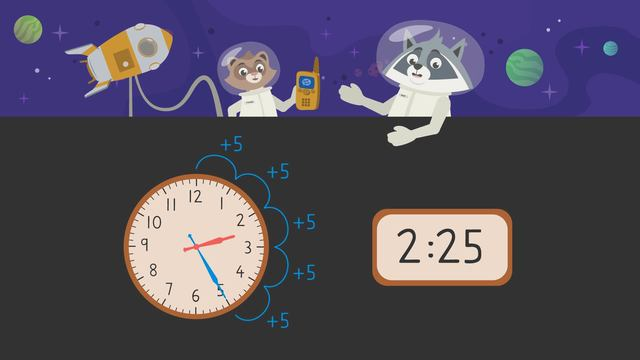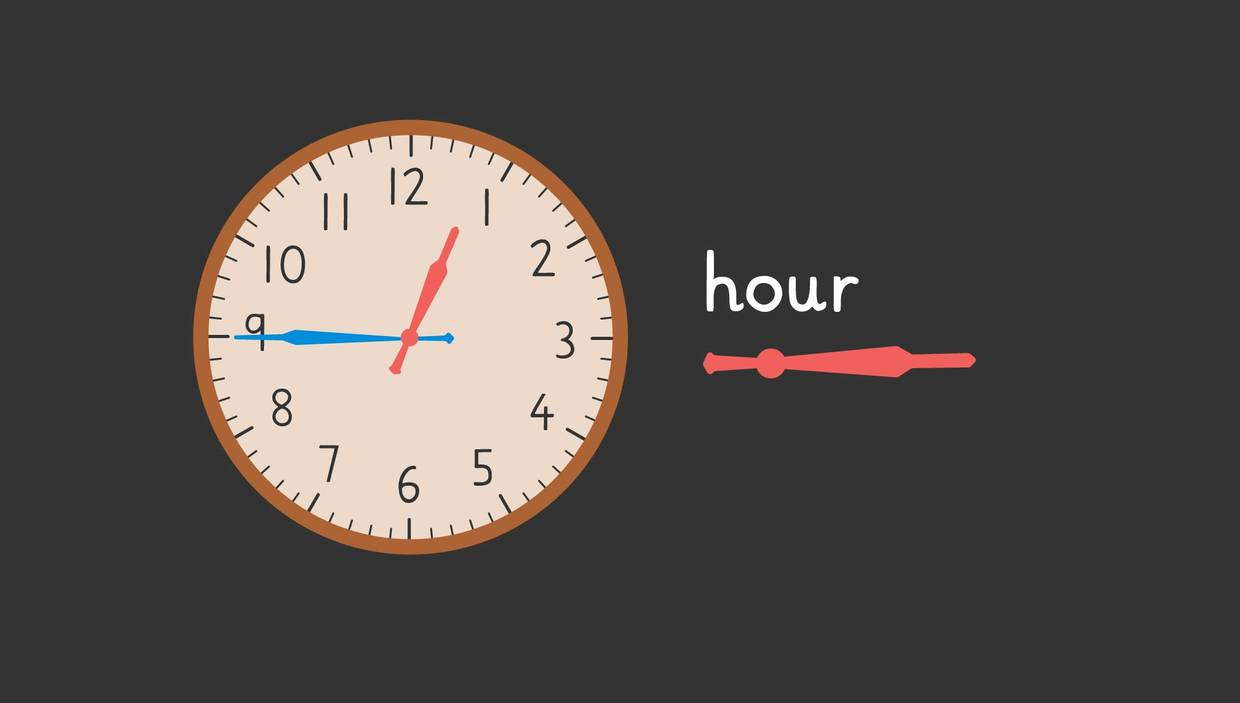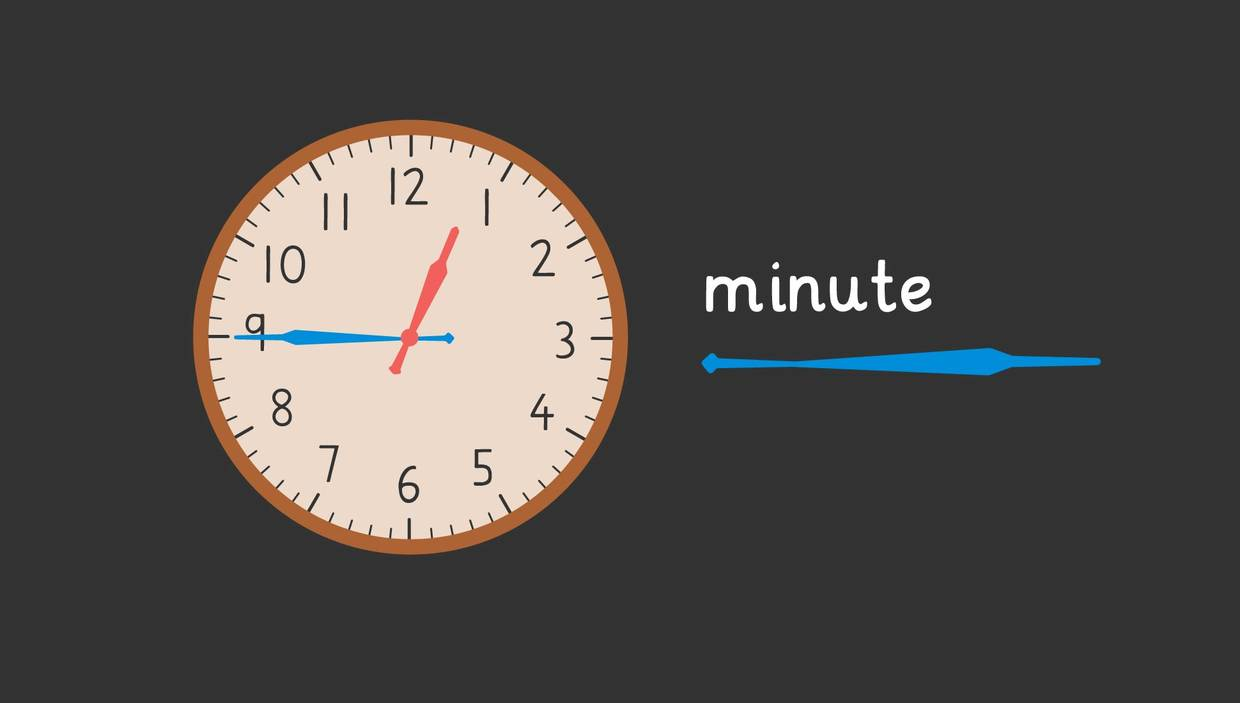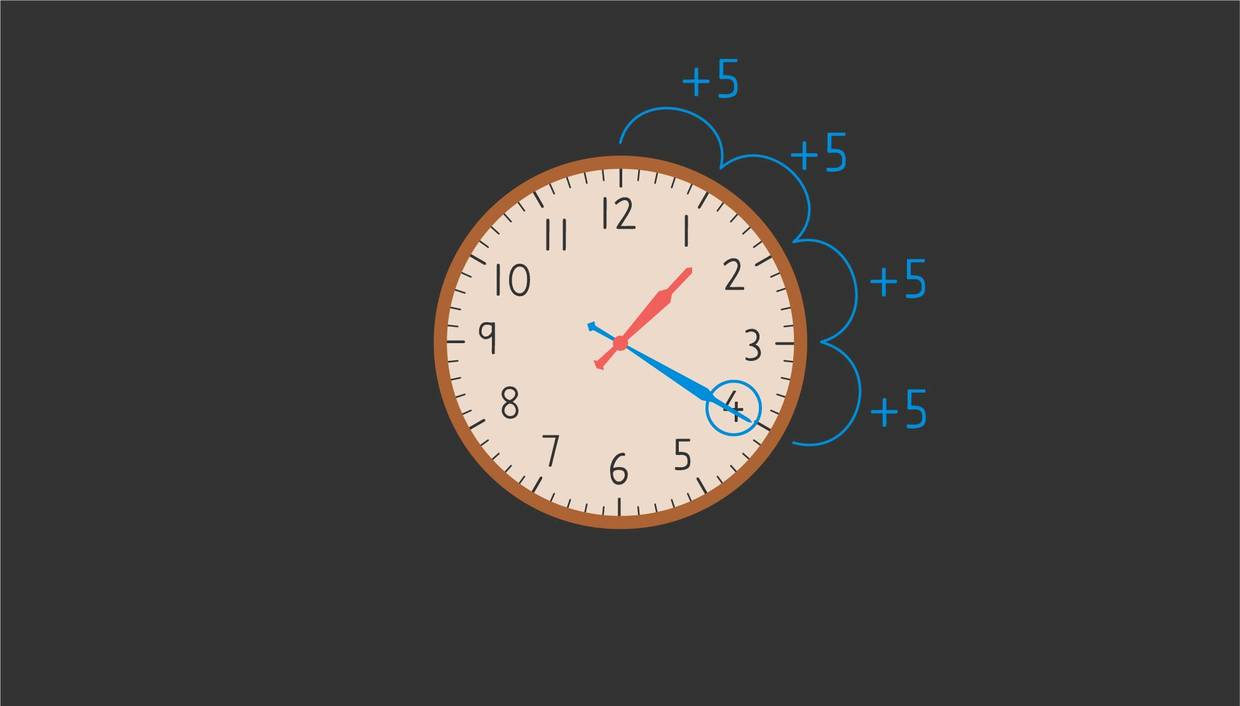# Telling Time to Five MinutesRating

Ø 3.5 / 11 ratings
The authorsTeam Digital
Telling Time to Five Minutes
CCSS.MATH.CONTENT.3.MD.A.1

## Telling Time to the Nearest 5 Minutes

How can you tell time using an analog clock? In this lesson we learn about skip counting as a strategy to help read analog clocks. Below we’ll explore more on how you can use what you know about skip counting and clocks to learn about how to tell time to the five minutes.

## The Clock – Hour and Minute Hands

When telling time to five minutes with an analog clock, we can use the arrows, often called hands, to help us figure out which is the hour and which is the minute on the clock. The hour hand is the shorter arrow, you might remember this because the word hour is short.While the minute hand is the longer arrow on the clock, you might remember this because the word minute is longer than the word hour.## Skip Counting – A Useful Method

Now that we’ve learned a bit about how to identify, or find the hour and minute hand, let’s learn how to read a clock using skip counting. What is skip counting? Skip counting is when you count forward by a specific amount. You can skip count forward by fives, tens, even hundreds. In this video we’re skip-counting by fives. Which means that we will skip count forward by five for every number that we see shown on the analog clock. This is also called telling time in a five minute interval.

## Telling Time to the Nearest 5 Minutes – Example

Below is an example of an analog clock. Can you use what you’ve learned about skip counting and identifying the hour and minute hand to read, or, tell what time it is?Start by finding the hour, you can do this by looking at the last number the hour hand has passed. The last number the hour hand passed here was one, so the hour is one. Next find the minute by skip-counting forward. In this video we skip-counted forward by fives, which means we count forward by five for each number represented on the analog clock. This can be helpful because each hour is shown five minutes apart on an analog clock. If we skip count by we can find the time to the nearest five minutes is 1:20. Did you also get that time? If not, take a look at the video again!

## Telling Time to the Nearest 5 Minutes – Summary

Remember when telling time to the nearest five minutes you should complete the following tasks in three steps:

Step one: Find the hour by looking at what number the hour hand last passed.
Step two: Find the minute by skip-counting.
Step three: Put the hour and minute together to find the time.

## Telling Time to the Nearest 5 Minutes – Additional Practice

Want more practice? At the end of the video, there are activities, such as exercises and games for continued practice of telling time to the nearest five minutes as well as worksheets with which you can exercise telling time to the nearest 5 minutes.

### TranscriptTelling Time to Five Minutes

Zuri and Freddie on behalf of the International Animals of Space Organization thank you for accepting the space exploration project. You should have received the tasks already, it's very important that you complete each task on time. I'll see you this afternoon for the video log check in. Good luck! "Let's take a look at the tasks!" "Do you think we'll see any aliens? I hope we see aliens!" "We have a problem though, I don't know how to use these clocks, they aren't like the ones I have at home." "Oh it's not that hard, we can skip count by fives to help us!" Telling Time to Five Minutes. Let's take a look at what Zuri and Freddie need to complete today. Zuri and Freddie need to start collecting space dust, but at what time? How can you read an analog clock to know the time? One way is, that we can skip count, or count forward by fives. Start by finding the hour. We can do this by finding the last number the short hand, or the hour hand last passed. Here the last number the hour hand passed was twelve, so the hour is twelve. Then skip count or count forward by fives to the minute hand, or the long hand. When we skip count on an analog clock we make hops around the clock, and every number we land on stands for five minutes. Count out loud with us! five, ten, fifteen, twenty, twenty-five, thirty, thirty-five, forty, forty-five. We skip counted by fives to the minute hand and got to forty-five so the minute is forty-five. If we put the hour and minute together we get the time, 12:45. After that, Zuri and Freddie need to polish the rings on Saturn. Let’s find out what time it is on the schedule by skip counting with fives. Start by finding the hour. Can you find the last number the hour hand passed? The hour is one. Then we can skip count by fives to get the minute. Skip count with me! Five, ten, fifteen, twenty. So the minute is twenty! If we put the hour and minute together our time is 1:20. Now Zuri and Freddie need to rearrange the stars to make a new constellation, but at what time? Remember, to start by finding the hour first by looking at the last number the hour hand past: What is the hour shown here? The hour is two. What about the minute? Let's skip count by fives to find the minute. What minute did you get to? If we skip count by fives we get the minute twenty-five. Let's put the hour and minute together, our time is 2:25. Zuri and Freddie are very busy in space, and need to count how many moons there are around Jupiter. What time do Zuri and Freddie need to do that? Remember to start by finding the hour. Which hour did you find? Next, skip count by fives to get to the minute hand. Then put the hour and minute together. What time did you get? The hour is three and the minute is fifty, so the time is 3:50 Last but not least Zuri and Freddie need to check in with the space commander with their walkie-talkies. Before we check in with the space commander, let's summarize. Remember when telling time to five minutes you start by finding the hour, or the last number the short hand has passed. Then you can find the minutes by skip counting or counting forward by fives. Once you have the hour and minute, you can put them together to find the time. "So, how was the mission? Did you see anything unusual?" "Everyone thinks Jupiter has 53 moons, but I counted 79!" "We completed everything on the list. It was pretty normal" "I was kind of hoping we'd see some aliens or something."

1 comment
1 comment
1.cool

From Lucas Scott Kerley, about 2 months ago

## Telling Time to Five Minutes exercise

Would you like to apply the knowledge you’ve learned? You can review and practice it with the tasks for the video Telling Time to Five Minutes .
• ### What time did Zuri and Freddie collect space dust?

Hints

Which hour has the short hand just passed? In this example it has just passed 10, so the hour is 10.

Remember to skip count by five from 12 to find the minutes. In this example the minute hand is at 6, so skip counting by five from 12 gets you to 30.

Solution
• Zuri and Freddie needed to collect space dust at 12:45.
• The last number the short hand passed was 12, so the hour is 12.
• Then, if you skip count by five from 12 to the long hand you get 45.
• When you put the hour and the minutes together, you get the time of 12:45!
• ### Can you match the times?

Hints

Look at each hour hand. Can this help you to find the correct times?

Which hour has the short hand just passed? In this example it has just passed 8, so the hour is 8.

Remember to skip count by five from 12 to find the minutes. In this example the minute hand is at 4, so skip counting by five from 12 gets you to 20.

Solution

Remember to find the hour first, then find the minutes.

For example, for the first question, the short hand has just passed 11, so the hour is 11.

To find the minutes, skip count by five from 12 until you reach the long hand. In this example the long hand is at 6, so skip counting by five from 12 will get you to 30. So the time is 11:30.

________________________________________________________

For the second question the short hand has just passed 9, so the hour is 9. The long hand is at 3, so we can skip count by five from 12 to 3, and we get to 15. The time is 9:15.

For the third question the short hand has just passed 4, so the hour is 4. The long hand is at 11, so we skip count by five from 12 all the way around until we get to 11. This gets us to 55 so the time is 4:55.

For the fourth question the short hand has just passed 7, so the hour is 7. The long hand is at 2, so we skip count by five from 12 until we get to 2. This gets us to 10 so the time is 7:10.

• ### What time did Zuri and Freddie need to complete their other tasks?

Hints

Pay close attention to which number the hour hand has just passed.

Have you skip counted by five from 12 to reach the long hand to find the minutes?

Solution

Remember to always check which number the short hand has just passed - this is the hour. For example, in the first pair the hour is 11. Then, skip count by five from 12 until you reach the long hand, these are the minutes. For this example, when you skip count by five from 12 to 9 you get 45. Lastly, you put the hour and minutes together to get the time of 11:45.

_____________________________________________________________________

• In the second pair the short hand is at 9 so the hour is 9. The minute hand is still at 12, there is no need to skip count by five, so the minute is 00. The time is 9:00 or 9 'o' clock.
• In the third pair the short hand has just passed 5 so the hour is 5. If you skip count by five from 12 around to 10 you get 50, so the time is **5:50.
• In the fourth pair, the short hand has just passed 6 so the hour is 6. If you skip count by five from 12 to 2 you get 10, so the time is 6:10.
• ### Can you write the correct times?

Hints

Make sure you:

• use digits only (not words for the times)
• put a colon between the hour and minutes
• don't use any spaces

What is the first step to read this clock? What is the second step?

Find the hour first by looking at the number the short hand has just passed.

Skip count by five from 12 to reach the long hand to find the minutes.

Solution

The example above shows us how to find the time on the first clock. The short hand has just passed 1 so the hour is 1.

The long hand is at 6 so we skip count by five from 12 to 6 and get 30, so the minutes are 30.

The time is 1:30.

Make sure you have written the time using digits with a colon between the hour and minutes.

________________________________________________________________________

• For the second clock the hour hand has just passed 3 so the hour is 3. Skip Counting by five from 12 to reach the long hand gets us to 20, so the time is 3:20.
• For the third clock the hour hand has just passed 5 so the hour is 5. Skip counting by five from 12 to reach the long hand gets us to 45, so the time is 5:45.
• For the fourth clock the hour hand has just passed 8 so the hour is 8. Skip counting by five from 12 to reach the long hand gets us to 55, so the time is 8:55.
• For the fifth clock the hour hand has just passed 10 so the hour is 10. Skip counting by five from 12 to reach the long hand gets us to 35, so the time is 10:35.
• For the sixth clock the hour hand has just passed 7 so the hour is 7. Skip counting in fives from 12 to reach the long hand gets us to 5, so the time is 7:05.
• ### How can you tell the time to five minutes?

Hints

Solution

We...

1. First, find the last number the short hand has passed. This is the hour.
2. Find where the long hand is pointing to.
3. Then, skip count by five from 12 around the clock to where the long hand is. The number you reach is the minutes.
4. Put the hour and the minutes together to tell the time!
____________________________________________________________

For example:

Start off by looking for the number that the short hand has just passed, this is the hour. In this example, the short hand has just passed 12. Next, you need to find the minutes. To do this, find the long hand then skip count by five from 12 until you reach the long hand. In this example, when you skip count by five you get 45. Lastly, you need to put the hour and the minutes together to tell the time, so the time would be 12:45.

• ### Can you find the incorrect times?

Hints

Have you figured out which hour the short hand has just passed?

Have you skip counted by five to the long hand to figure out the minutes?

Remember, only highlight the incorrect part.

Solution
• The first time was incorrect. Zuri and Freddie needed to skip count by fives one more time to get to 10:10.
• The second time was incorrect. Zuri and Freddie hadn't skip counted far enough by five to find the correct minute, 11:35.
• The third time was correct, well done Zuri and Freddie!
• The fourth time was incorrect. Zuri and Freddie did not find the correct hour; it should have been 5:15.